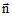# Definition of regions for an incomplete computation

## Introduction

The simplified or incomplete computation is a computation carried out under the following conditions:

• the computation is accomplished without taking into account of the current density in the structure
• the structure introduced in the electrolyte is represented by means of boundary conditions

## Volume region

Volume regions are gathered in the table below.

A volume region…

is a material region which enables the modeling…

electrolytic conducting of a conducting medium (resistivity ρ)
inactive of an insulating medium

## Face region

Face regions are gathered in the table below.

A face region… is a BC that enables one to impose…
electrochemical interface

conditions at the interface “electrolyte – cathode” by the intermediate of a polarization law

imposed potential

a potential (varying formula / spatial formula) (sacrificial anode)

imposed normal current density

a normal current density* (varying formula / spatial formula) (active anode)

Note: * The normalis directed from the face region towards the electrolyte.

## Line region

Line regions are gathered in the table below.

A line region … is a BC that enables one to impose …
imposed potential

a potential (varying formula / spatial formula) (sacrificial anode)

imposed normal current density

a radial current density* (varying formula / spatial formula) (active anode)

Note: * The normalis directed from the line region towards the electrolyte.

## Point region

Point regions are gathered in the table below.

A point region… is a BC that enables one to impose…
imposed potential a potential in a point